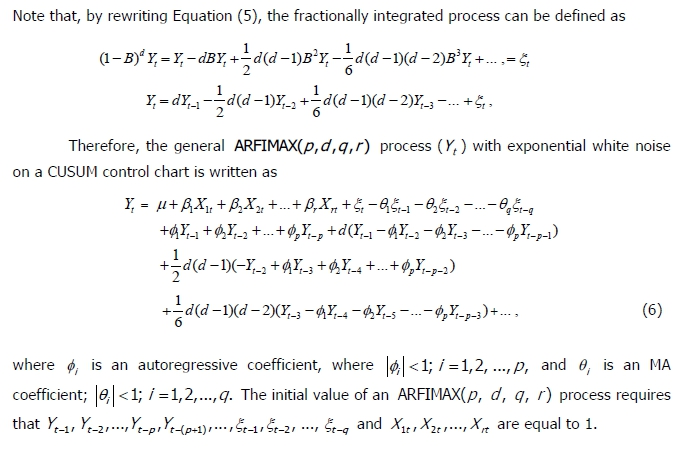# An Approximation to the Average Run Length on a CUSUM Control Chart with a Numerical Integral Equation Method for a Long-Memory ARFIMAX Model

• Wilasinee Peerajit Department of Applied Statistics, Faculty of Applied Science, King Mongkut’s University of Technology North Bangkok
Keywords: Exponential white noise, Numerical Integral Equation Method, ARFIMAX

### Abstract

The CUSUM control chart performance based on the out-of-control average run length (ARL) can rapidly detect small-to-moderate-sized changes in the process mean. The main goal of this study is to approximate the ARL using a numerical integral equation (NIE) method for detecting changes in the mean on a CUSUM control chart for a long-memory process with exponential white noise. The target long-process observations are derived from an autoregressive fractionally integrated moving-average (ARFIMA) model with explanatory variable X (ARFIMAX) by focusing on X. The NIE method is derived by using the Gauss-Legendre quadrature rule technique, which is applied by solving a system of linear equations base on integral equations. In addition, approximation by this method was numerically evaluated and the results compared with an ARL based on explicit formulas in terms of the percentage relative error. It was found that the proposed NIE method had comparable performance with the explicit-formula-based ARL method. Thus, the NIE method is a good alternative to using explicit formulas for constructing the ARL to detect changes in the mean for a long-memory process with exponential white noise on a CUSUM control chart.

### Downloads

Download data is not yet available.Published
2020-12-24
Section
Research Articles